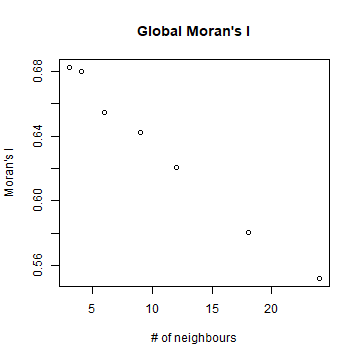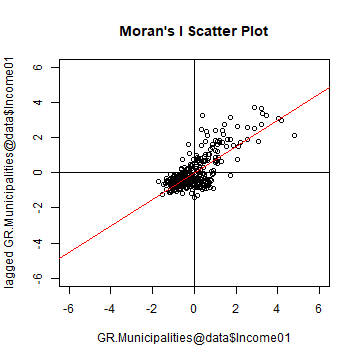# Introduction

## Exploratory Spatial Data Analysis (ESDA) & Spatial autocorrelation

Spatial autocorrelation is a method of Exploratory Spatial Data Analysis (ESDA). The latter set of methods allow for the study and understanding of the spatial distribution and spatial structure as well as they allow for detecting spatial dependence or autocorrelation in spatial data. More specifically, spatial autocorrelation is the correlation between the values of a single variable that is strictly due to the proximity of these values in geographical space by introducing a deviation from the assumption of independent observations of classical statistics (Griffith, 2003). The most common spatial autocorrelation indicators in the literature are: the Moran's I, the Geary's c, and the Getis' G.

## Moran's I

Moran's $$I$$ is one of the oldest statistics used to examine spatial autocorrelation. This global statistic was first proposed by Moran (1948, 1950). Later, Cliff and Ord (1973, 1981) present a comprehensive work on spatial autocorrelation and suggested a formula to calculate the $$I$$ which is now used in most textbooks and software: $$I = \frac{n}{W} \frac{\sum_{i=1}^{n} \sum_{j=1}^{n} w_{ij} z_i z_j} {\sum_{i=1}^{n} z_i^2}$$ where $$n$$ is number of observations, $$W$$ is the sum of the weights $$w_{ij}$$ for all pairs in the system, $$z_i=x_i - \bar{x}$$ where $$x$$ is the value of the variable at location $$i$$ and $$\bar{x}$$ the mean value of the variable in question (Eq. 5.2 Kalogirou, 2003).

The original implementation here (function moransI) allows only nearest neighbour weighting schemes. However, the function moransI.w allows for any weighting scheme if by means of a weighting matrix. Using the package lctools, the latter can be computed by the function w.matrix that currently supports a fixed (straight distance) and an adaptive (number of nearest neighbours) spatial kernels. Resampling and randomization null hypotheses have been tested following the discussion of Goodchild (1986, pp. 24-26).

# Exploring the data

The lctools package has some built in data to allow for practicing the various spatial analysis techniques. One of the datasets is GR.Municipalities. The latter is a SpatialPolygonsDataFrame that refers to the 325 Municipalities in Greece (Programme Kallikratis) in 2011. The descriptive data for each municipality include demographic and economic variables the source of which are the 2001 Census of Population and the General Secretariat for Information Systems, respectively.

library(lctools)
data(GR.Municipalities)
names(GR.Municipalities@data)

##   "OBJECTID"   "X"          "Y"          "Name"       "CodeELSTAT"
##   "PopM01"     "PopF01"     "PopTot01"   "UnemrM01"   "UnemrF01"
##  "UnemrT01"   "PrSect01"   "Foreig01"   "Income01"


To learn more about the above data set, try help(GR.Municipalities).

# Calculate the global Moran's I

To calculate the global Moran's I statistic one can: 1. make use of the function MoransI with three arguments: the coordinates of the observations, the number of nearest neighbours and the variable for which the statistic will be calculated for, or 2. calculate the weights matrix using the function w.matrix (arguments being the coordinates of the observations, the bandwidth -fixed or adaptive, and the weighting scheme) and then use the function moransI.w with two arguments: the weights matrix and the variable in question.

The coordinates refer to the geometric centro-ids of the municipalities in Greece. In the next two examples, the number of nearest neighbours is set to 6 and the straight distance bandwidth to 50 kilometres. The selected weights are binary ($$w_{ij}$$=1 for neighbours and =0 for non-neighbours. The variable to be analysed refers to the mean annual recorded income in 2001 (in Euros).

## Case 1: function MoransI

Coords <- cbind(GR.Municipalities@data$X, GR.Municipalities@data$Y)
bw <- 6
mI <- moransI(Coords,bw,GR.Municipalities@data$Income01) moran.table <- matrix(data=NA,nrow=1,ncol=6) col.names <- c("Moran's I", "Expected I", "Z resampling", "P-value resampling", "Z randomization", "P-value randomization") colnames(moran.table) <- col.names moran.table[1,1] <- mI$Morans.I
moran.table[1,2] <- mI$Expected.I moran.table[1,3] <- mI$z.resampling
moran.table[1,4] <- mI$p.value.resampling moran.table[1,5] <- mI$z.randomization
moran.table[1,6] <- mI$p.value.randomization  Using table formatting the results can be shown as follows: Moran's I Expected I Z resampling P-value resampling Z randomization P-value randomization 0.65441 -0.00309 22.27952 0 22.40946 0 ## Case 2: functions w.matrix and MoransI.w The above calculation can be repeated as follows: #adaptive kernel w.adaptive <- w.matrix(Coords,6, WType='Binary', family='adaptive') mI.adaptive <- moransI.w(GR.Municipalities@data$Income01, w.adaptive)


Using table formatting the results can be shown as follows:

Moran's I Expected I Z resampling P-value resampling Z randomization P-value randomization
0.65441 -0.00309 22.27952 0 22.40946 0

An example Moran's I calculation with a fixed spatial kernel and a 50 km radius as the bandwidth can be computed as follows:

#fixed kernel
w.fixed <- w.matrix(Coords, 50000, WType='Binary', family='fixed')

## The bandwidth  50000  will result  5  observations without neighbours.
## To avoid this you could set the bandwidth to at least:  146287.7

mI.fixed<- moransI.w(GR.Municipalities@data$Income01, w.fixed) mI.fixed <- t(as.numeric(as.matrix(mI.fixed[1:6]))) colnames(mI.fixed) <- col.names  The above bandwidth is such that 5 observations will have no neighbours. This is a result of a check that is printed out from the moransI.w function. These observations refer to isolated islands, such as Megisti east of Rhodes, which is 150 km away from its nearest neighbour. Using table formatting the results can be shown as follows: Moran's I Expected I Z resampling P-value resampling Z randomization P-value randomization 0.4813 -0.00309 16.50666 0 16.60292 0 The above results suggest a strong positive spatial autocorrelation that is statistically significant using either the randomization or resampling hypotheses (Cliff and Ord, 1973; 1981; Goodchild, 1986). In order to examine the sensitivity of the above results, one could try different bandwidth sizes (i.e. number of nearest neighbours). This can be done by either a loop or by using the function moransI.v that computes a number of Moran's I statistics with different kernel sizes of the same family and optionally shows a scatter plot with the Moran's I for each kernel size. bws <- c(3, 4, 6, 9, 12, 18, 24) moran <- moransI.v(Coords, bws, GR.Municipalities@data$Income01)The Moran's I along with significance tests for all spatial kernels are presented in the table below. The Moran's I statistics for the mean annual recorded income is above 0.5 in all cases suggesting a strong positive spatial autocorrelation. They are also statistically significant in all cases.

ID k Moran's I Expected I Z resampling P-value res. Z randomization P-value rand.
1 3 0.6823 -0.0031 16.4559 0 16.5519 0
2 4 0.6797 -0.0031 18.8569 0 18.9669 0
3 6 0.6544 -0.0031 22.2795 0 22.4095 0
4 9 0.6422 -0.0031 26.7714 0 26.9274 0
5 12 0.6206 -0.0031 29.9977 0 30.1725 0
6 18 0.5802 -0.0031 34.8110 0 35.0136 0
7 24 0.5523 -0.0031 38.8086 0 39.0342 0

# Local Moran's I

The next step is the calculation of local Moran $$I_i$$ using the function l.moransI, which creates a Moran's I Scatter Plot by default, as follows:

l.moran<-l.moransI(Coords,6,GR.Municipalities@data\$Income01)The object l.moran generated includes a plethora of results that can be plotted or mapped.

# References

Cliff, A.D., and Ord, J.K., 1973, Spatial autocorrelation (London: Pion).

Cliff, A.D., and Ord, J.K., 1981, Spatial processes: models and applications (London: Pion).

Goodchild, M. F., 1986, Spatial Autocorrelation. Catmog 47, Geo Books.

Griffith, D.A. (2003). Spatial autocorrelation and spatial filtering: gaining understanding through theory and scientific visualization. Berlin: Springer-Verlag.

Kalogirou, S., 2003, The Statistical Analysis and Modelling of Internal Migration Flows within England and Wales, PhD Thesis, School of Geography, Politics and Sociology, University of Newcastle upon Tyne, UK. \url{http://gisc.gr/?mdocs-file=1245&mdocs-url=false}

Kalogirou, S., 2015, Spatial Analysis: Methodology and Applications with R. [ebook] Athens: Hellenic Academic Libraries Link. ISBN: 978-960-603-285-1 (in Greek). \url{https://repository.kallipos.gr/handle/11419/5029?locale=en}

Moran, P.A.P., 1948, The interpretation of statistical maps, Journal of the Royal Statistics Society, Series B (Methodological), 10, 2, pp. 243 - 251.

Moran, P.A.P., 1950, Notes on continuous stochastic phenomena, Biometrika, 37, pp. 17 - 23.